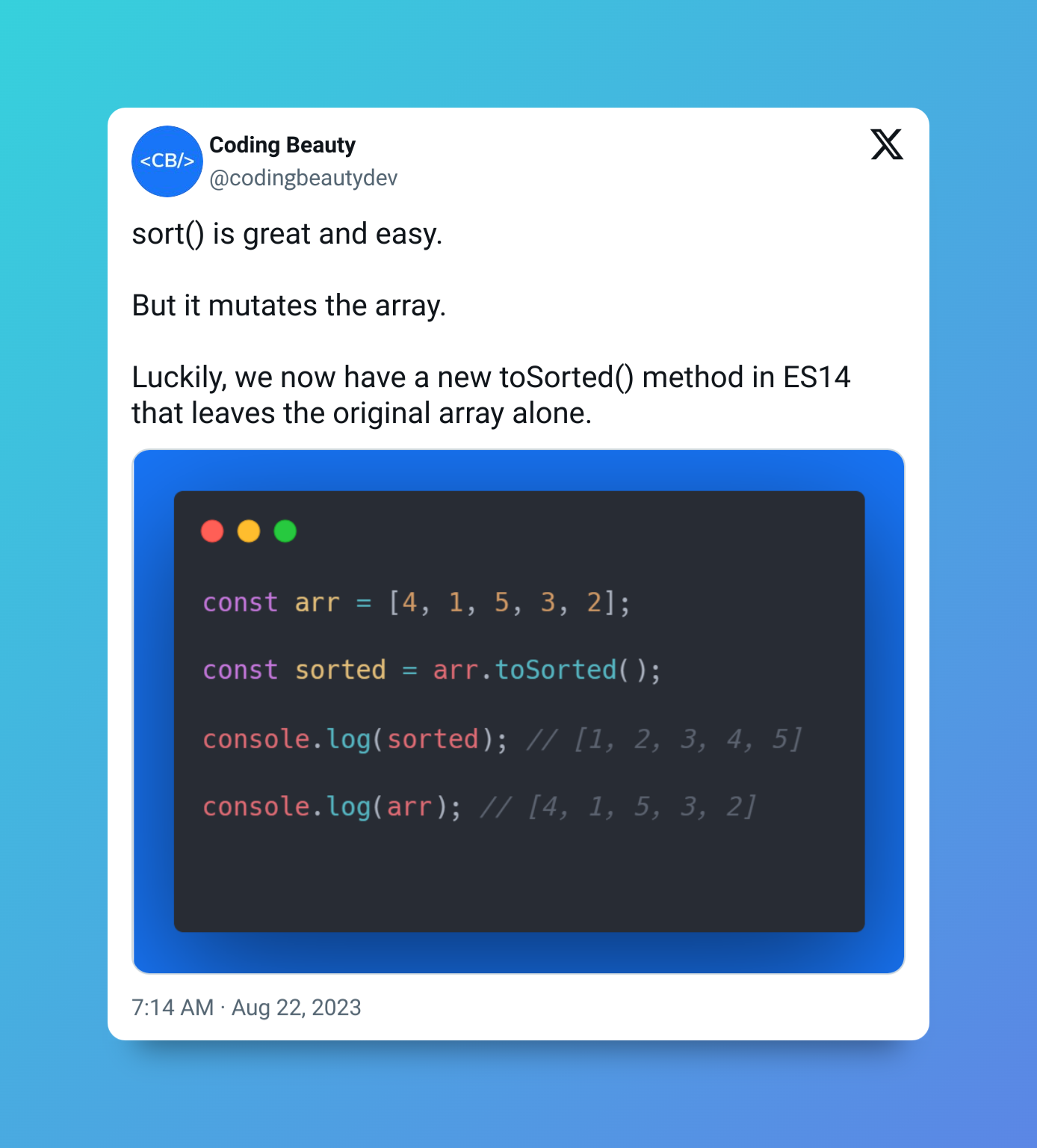# How to Subtract Seconds From a Date in JavaScript

Let’s learn how to easily subtract any number of seconds from a `Date` object in JavaScript.### 1. `Date``setSeconds()` and `getSeconds()` methods

To subtract seconds from a `Date`:

1. Call the `getSeconds()` method on the `Date` to get the number of seconds.
2. Subtract the seconds.
3. Pass the result of the subtraction to the `setSeconds()` method.

For example:

``````function subtractSeconds(date, seconds) {
date.setSeconds(date.getSeconds() - seconds);

return date;
}

// July 10, 2022 at 5:35:40 pm
const date = new Date('2022-07-10T17:35:40.000Z');

const newDate = subtractSeconds(date, 10);

// July 10, 2022 at 5:35:30 pm
console.log(newDate); // 2022-07-10T17:35:30.000Z
``````

Our `subtractSeconds()` function takes a `Date` object and the number of seconds to subtract as arguments. It returns the same `Date` object with the seconds subtracted.

The `Date` getSeconds() method returns a number between `0` and `59` that represents the seconds of a particular `Date`.

The `Date` setSeconds() method sets the seconds of a `Date` to a specified number.

If the seconds subtracted decrease the minute, hour, day, month, or year of the `Date` object, `setHours()` automatically updates the `Date` information to reflect this.

``````// July 10, 2022 at 5:20:00 pm
const date = new Date('2022-07-10T17:20:00.000Z');

date.setSeconds(date.getSeconds() - 130);

// July 10, 2022 at 5:17:50 pm
console.log(date); // 2022-07-10T17:17:50.000Z
``````

In this example, decreasing the seconds of the `Date` by `130` decreases the minutes by `3` and sets the seconds to `50`.

#### Avoiding side effects

The `setSeconds()` method mutates the `Date` object it is called on. This introduces a side effect into our `subtractSeconds()` function. To avoid modifying the passed, and create a pure function, make a copy of the `Date` and call `setSeconds()` on this copy, instead of the original.

``````function subtractSeconds(date, seconds) {
// make copy with Date() constructor
const dateCopy = new Date(date);

dateCopy.setSeconds(date.getSeconds() - seconds);

return dateCopy;
}

// July 10, 2022 at 5:35:40 pm
const date = new Date('2022-07-10T17:35:40.000Z');

const newDate = subtractSeconds(date, 10);

// July 10, 2022 at 5:35:30 pm
console.log(newDate); // 2022-07-10T17:35:30.000Z

// original not modified
console.log(date); // 2022-07-10T17:35:40.000Z
``````

Tip: Functions that don’t modify external state (i.e., pure functions) tend to be more predictable and easier to reason about, as they always give the same output for a particular input. This makes it a good practice to limit the number of side effects in your code.

### 2. date-fns `subSeconds()` function

Alternatively, we can use the `subSeconds()` function from the date-fns NPM package to quickly subtract seconds from a `Date`. It works similarly to our pure `subtractSeconds()` function.

``````import { subSeconds } from 'date-fns';

// July 10, 2022 at 5:35:40 pm
const date = new Date('2022-07-10T17:35:40.000Z');

const newDate = subSeconds(date, 10);

// July 10, 2022 at 5:35:30 pm
console.log(newDate); // 2022-07-10T17:35:30.000Z

// original not modified
console.log(date); // 2022-07-10T17:35:40.000Z
``````

## Every Crazy Thing JavaScript Does

A captivating guide to the subtle caveats and lesser-known parts of JavaScript.